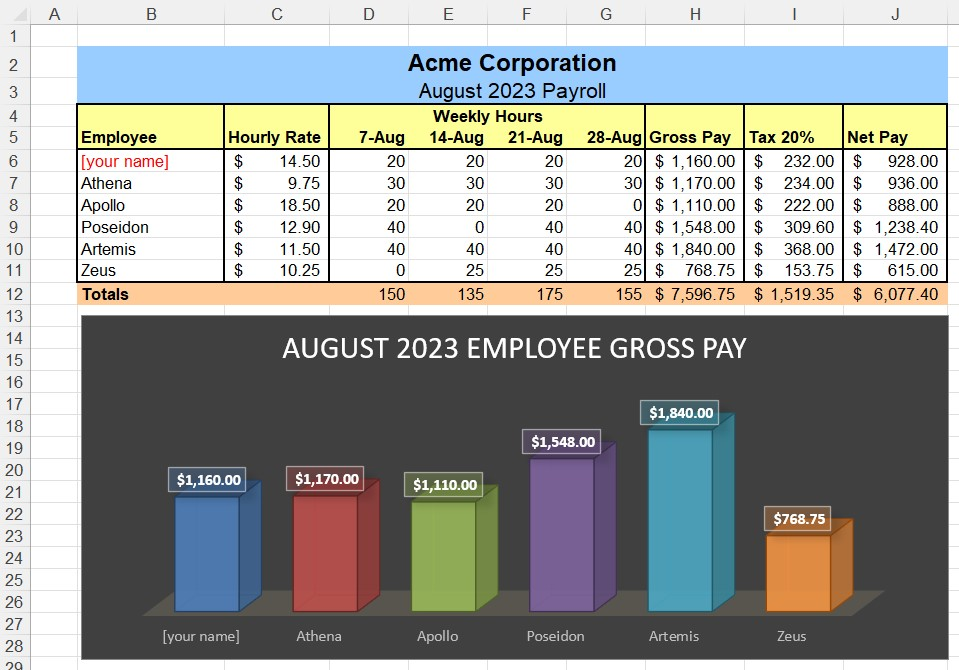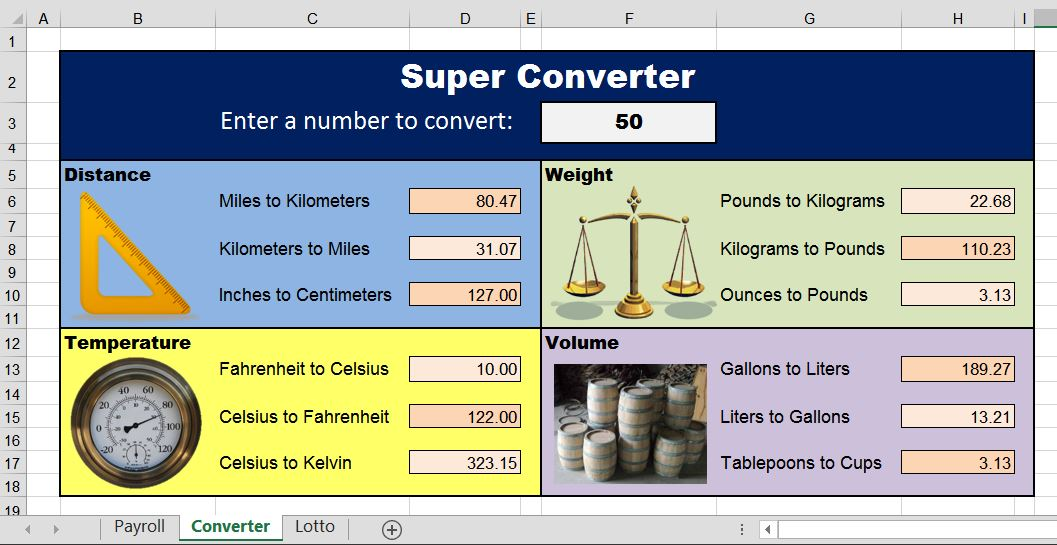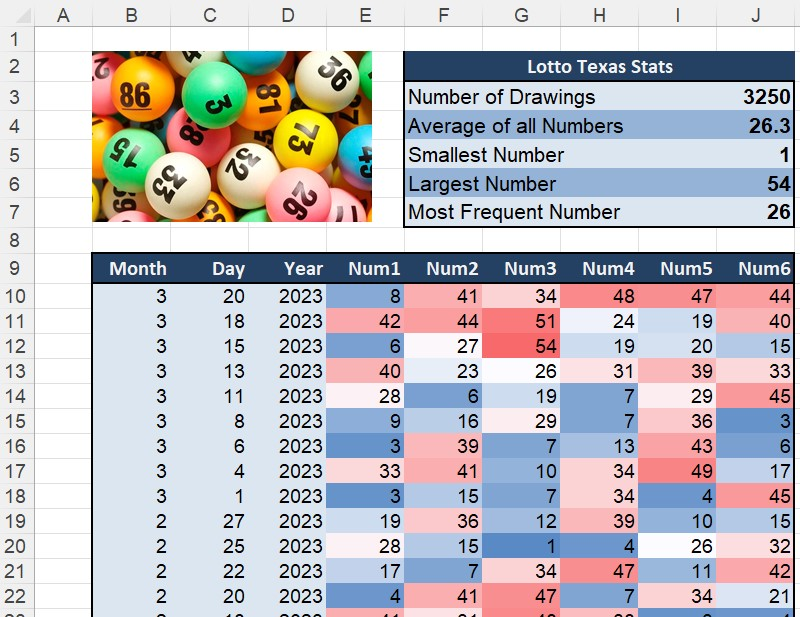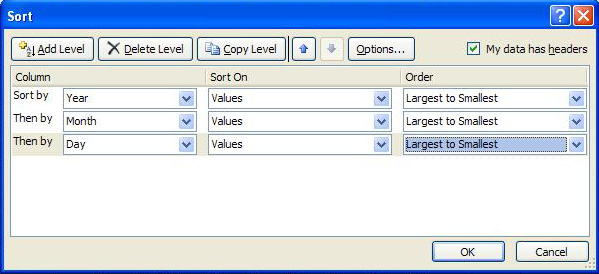Homework 4 - Excel

Instructions:

• Using Microsoft Excel, create a single file with three sheets (named Payroll, Converter, and Lotto)
• Follow the instructions below for creating each sheet
• Name the file Your_Name_HW3  (The .xls or .xlsx file extension is automatically added)
• Submit your .xlsx file on eCampus.
• Introduction to Excel and formulas videos:   Part 1    Part 2    Homework hints

IMPORTANT: The spreadsheets you create must contain formulas as specified in the instructions (40% of the grade).  Follow the instructions in each section on which cells contain formulas.

1. Payroll Sheet• For this sheet, duplicate the formatting (fonts, colors, lines) as closely as possible
• Copy the employee names, hourly rates, and weekly hours data as shown.  Note that the first employee should be Your Name.

• The Gross Pay column are formulas that multiply the employee's Hourly Rate by their Weekly Hours
For example, cell H6 contains this formula:      =C6 * Sum(D6:G6)
• The Tax 20% column is are formulas that multiply the employee's Gross Pay by 20%
For example, cell I6 contains this formula:       =H6*0.2
• The Net Pay column is are formulas that subtract the employee's Tax from their Gross Pay
For example, cell J6 formula is     =H6-I6
• Totals are formulas that sum each column

• Create a chart showing the gross pay for each employee.  Hint:  (1) Select the employee names, (2) press and hold the Ctrl key, (3) select the gross pay amounts, (4) insert the column chart.
• Rename this sheet to Payroll (look at bottom of spreadsheet).  Rename the other sheets to Conversion and Lotto.

2. Converter Sheet• This sheet has a cell (F3) for the user to enter a number.  It will perform the 12 conversions as shown using the convert function.

• Each of the 12 conversions needs a formula.  Here are a couple to get you started:
Cell D6 contains this formula:
=CONVERT(F3, "mi", "km")
Cell H6 contains this formula:     =CONVERT(F3, "lbm", "kg")

• Format the sheet with colors, lines, shading, and pictures, but you do not need to duplicate the exact formatting of the table shown.

• Use Google or Excel Help to find the abbreviations for the other conversions.  Note that in old versions of Excel (before 2007), you may need to add the Analysis Toolpak for the Convert function to work.

3. Lotto Sheet• This sheet should contain all the Lotto Texas drawing results since the game began in 1992 (over 2000 drawings).  You may download the data from their web site:  Download Download Lotto Texas Past Winning Numbers.  The data is in a CSV file that will open with Excel.  You will need to copy and paste the data into your Homework spreadsheet.

• Write formulas to calculate the Number of Drawings (count function), Average of all Balls (average), Smallest Ball (min), Largest Ball (max), and the most frequently occurring ball (mode).  Note: Your results will be different since you will be downloading the lotto data file at a later date.

• Sort the data/drawings from newest to oldest.  Select all the data, click Excel's Data tab, and select Sort.  In the Sort window shown below, sort by Year, Month, and Day from Largest to Smallest.• Format the sheet with colors, lines, shading, and a picture, but you do not need to duplicate the exact formatting of the table shown.